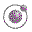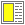infinite-ex.scm - A more concrete version of the expression from above. Lecture 5 - slide 6 : 26Program 1

```(define (infinite-calculation)
(infinite-calculation))

((lambda (x) 1) (infinite-calculation))```